# Ohm’s Law Formula

The relationship between Voltage, Current and Resistance in any DC electrical circuit was firstly discovered by the German physicist Georg Ohm.Georg Ohm found that, at a constant temperature, the electrical current flowing through a fixed linear resistance is directly proportional to the voltage applied to it, and also inversely proportional to the resistance. This relationship between the Voltage, Current and Resistance forms the basis of Ohm’s Law and is shown below.

### Ohm’s Law Relationship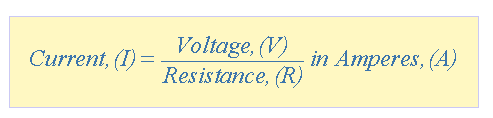By knowing any two values of the Voltage, Current or Resistance quantities we can use Ohm’s Law to find the third missing value. Ohm’s Law is used extensively in electronics formulas and calculations so it is “very important to understand and accurately remember these formulas”.

### To find the Voltage, ( V )

[ V = I x R ]      V (volts) = I (amps) x R (Ω)

### To find the Current, ( I )

[ I = V ÷ R ]      I (amps) = V (volts) ÷ R (Ω)

### To find the Resistance, ( R )

[ R = V ÷ I ]      R (Ω) = V (volts) ÷ I (amps)

It is sometimes easier to remember this Ohm’s law relationship by using pictures. Here the three quantities of VI and R have been superimposed into a triangle (affectionately called the Ohm’s Law Triangle) giving voltage at the top with current and resistance below. This arrangement represents the actual position of each quantity within the Ohm’s law formulas.

### Ohm’s Law TriangleTransposing the standard Ohm’s Law equation above will give us the following combinations of the same equation: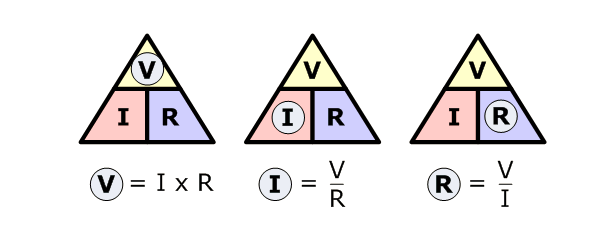Then by using Ohm’s Law we can see that a voltage of 1V applied to a resistor of 1Ω will cause a current of 1A to flow and the greater the resistance value, the less current that will flow for a given applied voltage. Any Electrical device or component that obeys “Ohm’s Law” that is, the current flowing through it is proportional to the voltage across it ( I α V ), such as resistors or cables, are said to be “Ohmic” in nature, and devices that do not, such as transistors or diodes, are said to be “Non-ohmic” devices.

## Electrical Power in Circuits

Electrical Power, ( P ) in a circuit is the rate at which energy is absorbed or produced within a circuit. A source of energy such as a voltage will produce or deliver power while the connected load absorbs it. Light bulbs and heaters, for example, absorb electrical power and convert it into either heat, or light, or both. The higher their value or rating in watts the more electrical power they are likely to consume.

The quantity symbol for power is P and is the product of voltage multiplied by the current with the unit of measurement is the Watt ( W ). Prefixes are used to denote the various multiples or sub-multiples of a watt, such as milliwatts (mW = 10-3W) or kilowatts (kW = 103W).

Then by using Ohm’s law and substituting for the values of VI and R the formula for electrical power can be found at:

### To find the Power (P)

[ P = V x I ]      P (watts) = V (volts) x I (amps)

Also,

[ P = V2 ÷ R ]      P (watts) = V2 (volts) ÷ R (Ω)

Also,

[ P = I2 x R ]      P (watts) = I2 (amps) x R (Ω)

Again, the three quantities have been superimposed into a triangle this time called a Power Triangle with power at the top and current and voltage at the bottom. Again, this arrangement represents the actual position of each quantity within the Ohm’s law power formulas.

### The Power Triangleand again, transposing the basic Ohm’s Law equation above for power gives us the following combinations of the same equation to find the various individual quantities: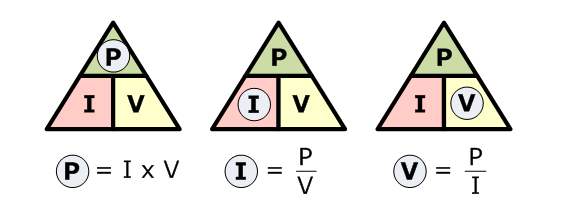So we can see that there are three possible formulas for calculating electrical power in a circuit. If the calculated power is positive, (+P) in value for any formula the component absorbs the power, that is it is consuming or using power. But if the calculated power is negative, (–P) in value the component produces or generates power, in other words, it is a source of electrical power such as batteries and generators.

## Electrical Power Rating

Electrical components are given a “power rating” in watts that indicate the maximum rate at which the component converts the electrical power into other forms of energy such as heat, light or motion. For example, a 1/4W resistor, a 100W light bulb etc.

Electrical devices convert one form of power into another. So for example, an electrical motor will convert electrical energy into a mechanical force, while an electrical generator converts mechanical force into electrical energy. A light bulb converts electrical energy into both light and heat.

Also, we now know that the unit of power is the WATT, but some electrical devices such as electric motors have a power rating in the old measurement of “Horsepower” or hp. The relationship between horsepower and watts is given as 1hp = 746W. So for example, a two-horsepower motor has a rating of 1492W, (2 x 746) or 1.5kW.

## Ohm’s Law Pie Chart

To help us understand the relationship between the various values a little further, we can take all of the Ohm’s Law equations from above for finding VoltageCurrentResistance and of course Power and condense them into a simple Ohm’s Law pie chart for use in AC and DC circuits and calculations as shown.

### Ohm’s Law Pie Chart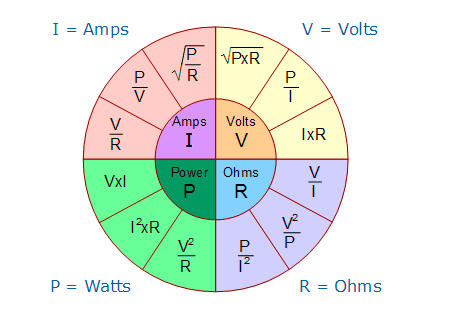As well as using the Ohm’s Law Pie Chart shown above, we can also put the individual Ohm’s Law equations into a simple matrix table as shown for easy reference when calculating an unknown value.

### Ohm’s Law Matrix Table## Ohm’s Law Example No1

For the circuit shown below find the Voltage (V), the Current (I), the Resistance (R) and the Power (P).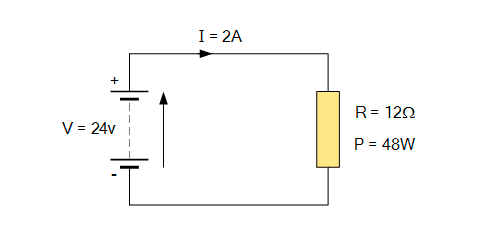Voltage   [ V = I x R ] = 2 x 12Ω = 24V

Current   [ I = V ÷ R ] = 24 ÷ 12Ω = 2A

Resistance   [ R = V ÷ I ] = 24 ÷ 2 = 12 Ω

Power   [ P = V x I ] = 24 x 2 = 48W

Power within an electrical circuit is only present when BOTH voltage and current are present. For example, in an open-circuit condition, voltage is present but there is no current flow I = 0 (zero), therefore V x 0 is 0 so the power dissipated within the circuit must also be 0. Likewise, if we have a short-circuit condition, current flow is present but there is no voltage V = 0, therefore 0 x I = 0 so again the power dissipated within the circuit is 0.

As electrical power is the product of V x I, the power dissipated in a circuit is the same whether the circuit contains high voltage and low current or low voltage and high current flow. Generally, electrical power is dissipated in the form of Heat (heaters), Mechanical Work such as motors, Energy in the form of radiated (Lamps) or as stored energy (Batteries).

## Electrical Energy in Circuits

Electrical Energy is the capacity to do work, and the unit of work or energy is the jouleJ ). Electrical energy is the product of power multiplied by the length of time it was consumed. So if we know how much power, in Watts is being consumed and the time, in seconds for which it is used, we can find the total energy used in watt-seconds. In other words, Energy = power x time and Power = voltage x current. Therefore electrical power is related to energy and the unit given for electrical energy is the watt-seconds or joules.Electrical power can also be defined as the rate of by which energy is transferred. If one joule of work is either absorbed or delivered at a constant rate of one second, then the corresponding power will be equivalent to one watt so power can be defined as “1Joule/sec = 1Watt”. Then we can say that one watt is equal to one joule per second and electrical power can be defined as the rate of doing work or the transferring of energy.

### Electrical Power and Energy Triangleor to find the various individual quantities: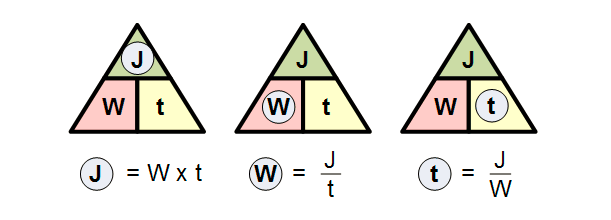We said previously that electrical energy is defined as being watts per second or joules. Although electrical energy is measured in Joules it can become a very large value when used to calculate the energy consumed by a component.

For example, if a 100 watt light bulb is left-“ON” for 24 hours, the energy consumed will be 8,640,000 Joules (100W x 86,400 seconds), so prefixes such as kilojoules (kJ = 103J) or megajoules (MJ = 106J) are used instead and in this simple example, the energy consumed will be 8.64MJ (megajoules).

But dealing with joules, kilojoules or megajoules to express electrical energy, the maths involved can end up with some big numbers and lots of zeroes, so it is much easier to express electrical energy consumed in Kilowatt-hours.

If the electrical power consumed (or generated) is measured in watts or kilowatts (thousands of watts) and the time is measured in hours not seconds, then the unit of electrical energy will be the kilowatt-hours,(kWhr). Then our 100 watts light bulb above will consume 2,400-watt-hours or 2.4kWhr, which is much easier to understand the 8,640,000 joules.

1 kWh is the amount of electricity used by a device rated at 1000 watts in one hour and is commonly called a “Unit of Electricity”. This is what is measured by the utility meter and is what we as consumers purchase from our electricity suppliers when we receive our bills.

Kilowatt-hours are the standard units of energy used by the electricity meter in our homes to calculate the amount of electrical energy we use and therefore how much we pay. So if you switch ON an electric fire with a heating element rated at 1000 watts and left it on for 1 hour you will have consumed 1 kWhr of electricity. If you switched on two electric fires each with 1000 watt elements for half an hour the total consumption would be exactly the same amount of electricity – 1kWhr.

So, consuming 1000 watts for one hour uses the same amount of power as 2000 watts (twice as much) for half an hour (half the time). Then for a 100 watt light bulb to use 1 kWhr or one unit of electrical energy, it would need to be switched on for a total of 10 hours (10 x 100 = 1000 = 1kWhr).

Now that we know what is the relationship between voltage, current, and resistance in a circuit, in the next tutorial relating to DC Circuits, we will look at the Standard Electrical Units used in electrical and electronic engineering to enable us to calculate these values and see that each value can be represented by either multiples or sub-multiples of the standard unit.